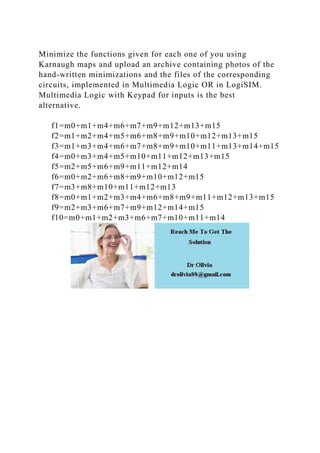Seu SlideShare está sendo baixado. ×

# Minimize the functions given for each one of you using Karnaugh maps.docx

Anúncio
Anúncio
Anúncio
Anúncio
Anúncio
Anúncio
Anúncio
Anúncio
Anúncio
Anúncio
AnúncioCarregando em…3
×

1 de 1 Anúncio

# Minimize the functions given for each one of you using Karnaugh maps.docx

Minimize the functions given for each one of you using Karnaugh maps and upload an archive containing photos of the hand-written minimizations and the files of the corresponding circuits, implemented in Multimedia Logic OR in LogiSIM. Multimedia Logic with Keypad for inputs is the best alternative.

f1=m0+m1+m4+m6+m7+m9+m12+m13+m15      f2=m1+m2+m4+m5+m6+m8+m9+m10+m12+m13+m15      f3=m1+m3+m4+m6+m7+m8+m9+m10+m11+m13+m14+m15      f4=m0+m3+m4+m5+m10+m11+m12+m13+m15      f5=m2+m5+m6+m9+m11+m12+m14      f6=m0+m2+m6+m8+m9+m10+m12+m15      f7=m3+m8+m10+m11+m12+m13      f8=m0+m1+m2+m3+m4+m6+m8+m9+m11+m12+m13+m15      f9=m2+m3+m6+m7+m9+m12+m14+m15      f10=m0+m1+m2+m3+m6+m7+m10+m11+m14
.

Minimize the functions given for each one of you using Karnaugh maps and upload an archive containing photos of the hand-written minimizations and the files of the corresponding circuits, implemented in Multimedia Logic OR in LogiSIM. Multimedia Logic with Keypad for inputs is the best alternative.

f1=m0+m1+m4+m6+m7+m9+m12+m13+m15      f2=m1+m2+m4+m5+m6+m8+m9+m10+m12+m13+m15      f3=m1+m3+m4+m6+m7+m8+m9+m10+m11+m13+m14+m15      f4=m0+m3+m4+m5+m10+m11+m12+m13+m15      f5=m2+m5+m6+m9+m11+m12+m14      f6=m0+m2+m6+m8+m9+m10+m12+m15      f7=m3+m8+m10+m11+m12+m13      f8=m0+m1+m2+m3+m4+m6+m8+m9+m11+m12+m13+m15      f9=m2+m3+m6+m7+m9+m12+m14+m15      f10=m0+m1+m2+m3+m6+m7+m10+m11+m14
.

Anúncio
Anúncio

### Minimize the functions given for each one of you using Karnaugh maps.docx

1. 1. Minimize the functions given for each one of you using Karnaugh maps and upload an archive containing photos of the hand-written minimizations and the files of the corresponding circuits, implemented in Multimedia Logic OR in LogiSIM. Multimedia Logic with Keypad for inputs is the best alternative. f1=m0+m1+m4+m6+m7+m9+m12+m13+m15 f2=m1+m2+m4+m5+m6+m8+m9+m10+m12+m13+m15 f3=m1+m3+m4+m6+m7+m8+m9+m10+m11+m13+m14+m15 f4=m0+m3+m4+m5+m10+m11+m12+m13+m15 f5=m2+m5+m6+m9+m11+m12+m14 f6=m0+m2+m6+m8+m9+m10+m12+m15 f7=m3+m8+m10+m11+m12+m13 f8=m0+m1+m2+m3+m4+m6+m8+m9+m11+m12+m13+m15 f9=m2+m3+m6+m7+m9+m12+m14+m15 f10=m0+m1+m2+m3+m6+m7+m10+m11+m14